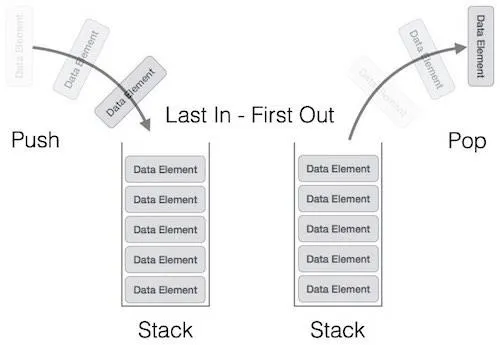# Programming - C Stacks using ArraysHello again my Friends! Today we will talk about Stacks in C, implemented using Arrays. We will talk about basic inserting and deleting, and off course printing. The post will look almost identical to the queue's one. So, without further do, let's get started.

# Stack Array Implementation:

A Stack is a Data Structure that implements the LIFO (last in first out) way of getting the data in and out. So, we insert on top and get from top. Exactly the same way as we would stack books. We can't get a book that is not on the top, without getting the others on top of it before. We define a stack again as a struct that has to have:

• an static Array that contains the Stack Data
• the top index of the Stack Array, where the last Item sits

We could also include capacity, if we make it pseudodynamic.

To keep it simple I will only talk about Integer Arrays. So, an struct of an Stack will look like this:

``typedef struct Stack{``
`` int s[N];``
`` int top;``
``}Stack;``

The top will be initialized as -1, that means the stack is empty.

The functions that use a Stack will have an struct variable of type Stack and we will use this variable for doing stuff like adding, removing an Item or printing the Stack. The adding and removing need the address so we have a stack pointer, and for printing we will use the same trick as in Queues passing the Stack variable only without address and calling removing as long as there are items.

For adding we have to check if stack is full that means we can't add a new Item. Afterwards, we simply increment the top index and insert the new value to the top index. So, our Code looks like this:

``void push(Stack *S, int val){``
`` // check if full``
`` if((S->top + 1) == N){``
``     printf("Stack is Full!\n");``
``     return;``
`` }``
`` // simply increment rear index``
`` S->top++;``
`` // add item to the top of Stack``
`` S->s[S->top] = val;``
``}``

# Removing (Pop):

When removing an item we have to check if stack is empty(that means we return -1), store the value in top index in a temporary variable, decrease the top index and return the value. In generall the stack is easy when using arrays.

So, our Code looks like this:

``int pop(Stack *S){``
`` int val;``
`` // check if empty``
`` if(S->top == -1){``
``     return -1;``
`` }``
`` // save value to temp``
`` val = S->s[S->top];``
`` // decrease top index``
`` S->top--;``
`` //return the value ``
`` return val; ``
``}``

# Printing:

Last but not least comes printing. We will pass the variable and not the address and call the pop function as long as there are items. This way it's pretty simple, but you could also use a for loop from top to 0 decreasing the loop counter.

So, our this is our Code:

``void print(Stack S){``
`` int val;``
`` // check if empty``
`` if(S.top == -1){``
``     printf("Stack is Empty!\n");``
``     return;``
`` }``
`` // call pop as long as there are items``
`` while((val = pop(&S)) != -1){``
``     printf("%d ", val);``
`` }   ``
`` printf("\n");``
``}``

# Code:

Last for today here a Code that uses those functions so that you know how to set them up. Again, make sure to include the define N statement.

``int main(){``
`` //initialize Stack``
`` Stack S;``
`` S.top = -1;``
`` print(S);``
`` // add 3 to stack``
`` push(&S, 5);``
`` print(S);``
`` // add 7 to stack``
`` push(&S, 10);``
`` print(S);``
`` // add 2 to stack``
`` push(&S, 2);``
`` print(S);``
`` // remove from stack``
`` int val;``
`` val = pop(&S);``
`` if(val == -1){``
``     printf("Queue is Empty!\n");``
`` }``
`` else{``
``     printf("%d\n", val);``
`` }``
`` print(S);   ``
``}``

So, that was the end of todays Tutorial. Hope you enjoy it.

Next 2 posts will be the exact same thing that we did for Queues and Stacks, but using Linked Lists.

H2
H3
H4
3 columns
2 columns
1 column